# NCERT Solutions for Class 9 Science Chapter 9

NCERT Solutions for Class 9 Science Chapter 9 – Force and Laws of Motion, contains solutions to various questions in Exercises for Chapter 9. Force and Laws of Motion Class 9 NCERT Solutions have been explained in a simple and easy to understand manner. We are providing all subjects which can be accessed by clicking here.

## Download NCERT Solutions for Class 9 Science Chapter 9 – Force and Laws of Motion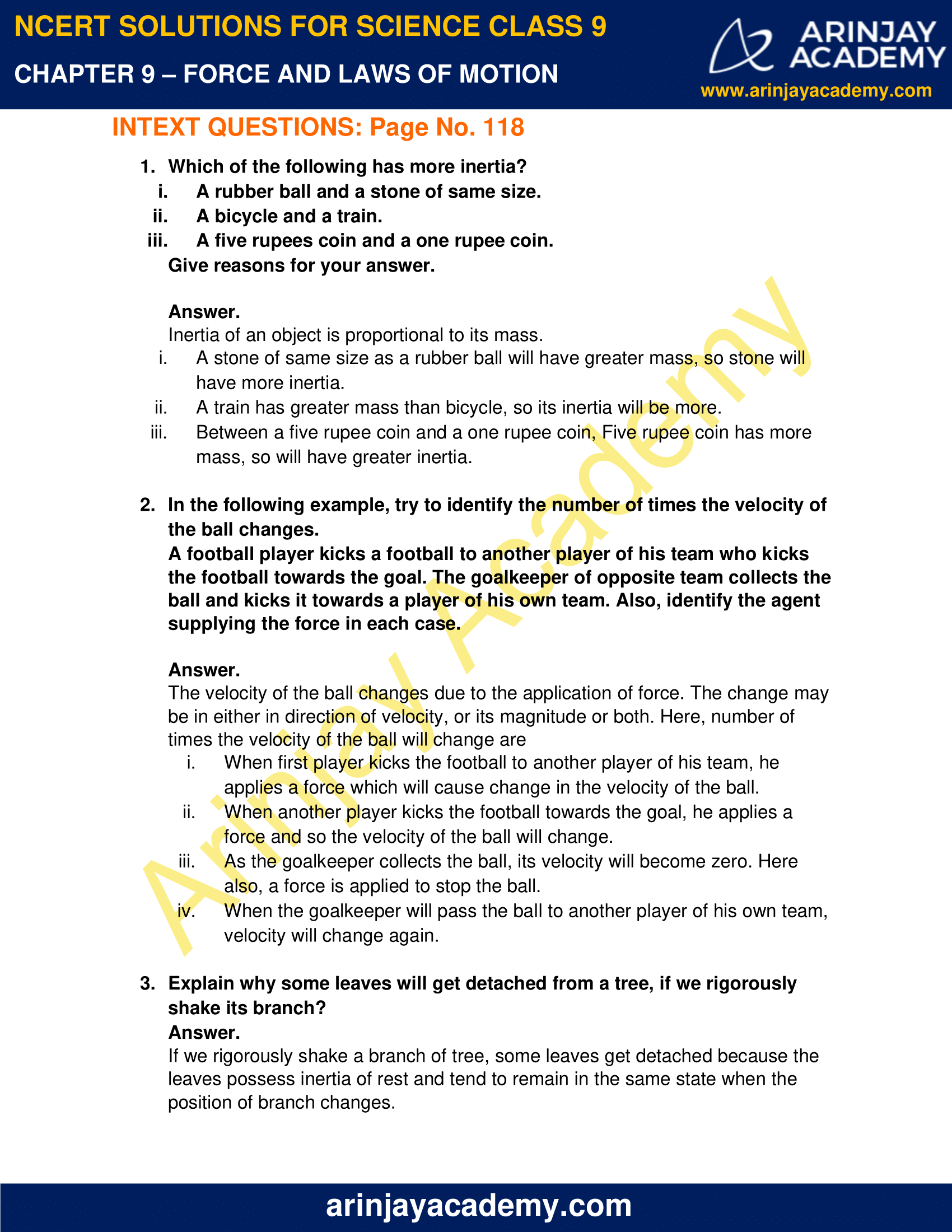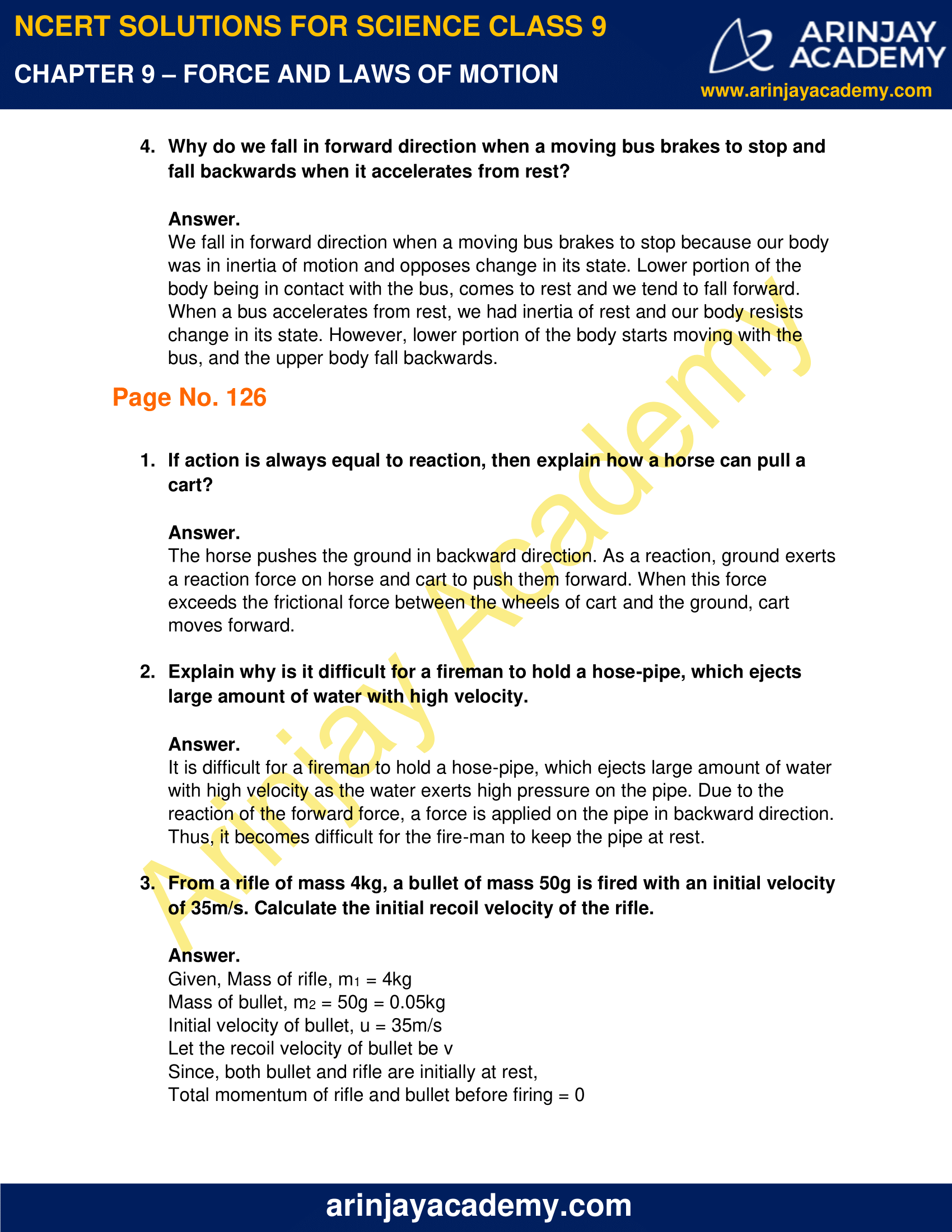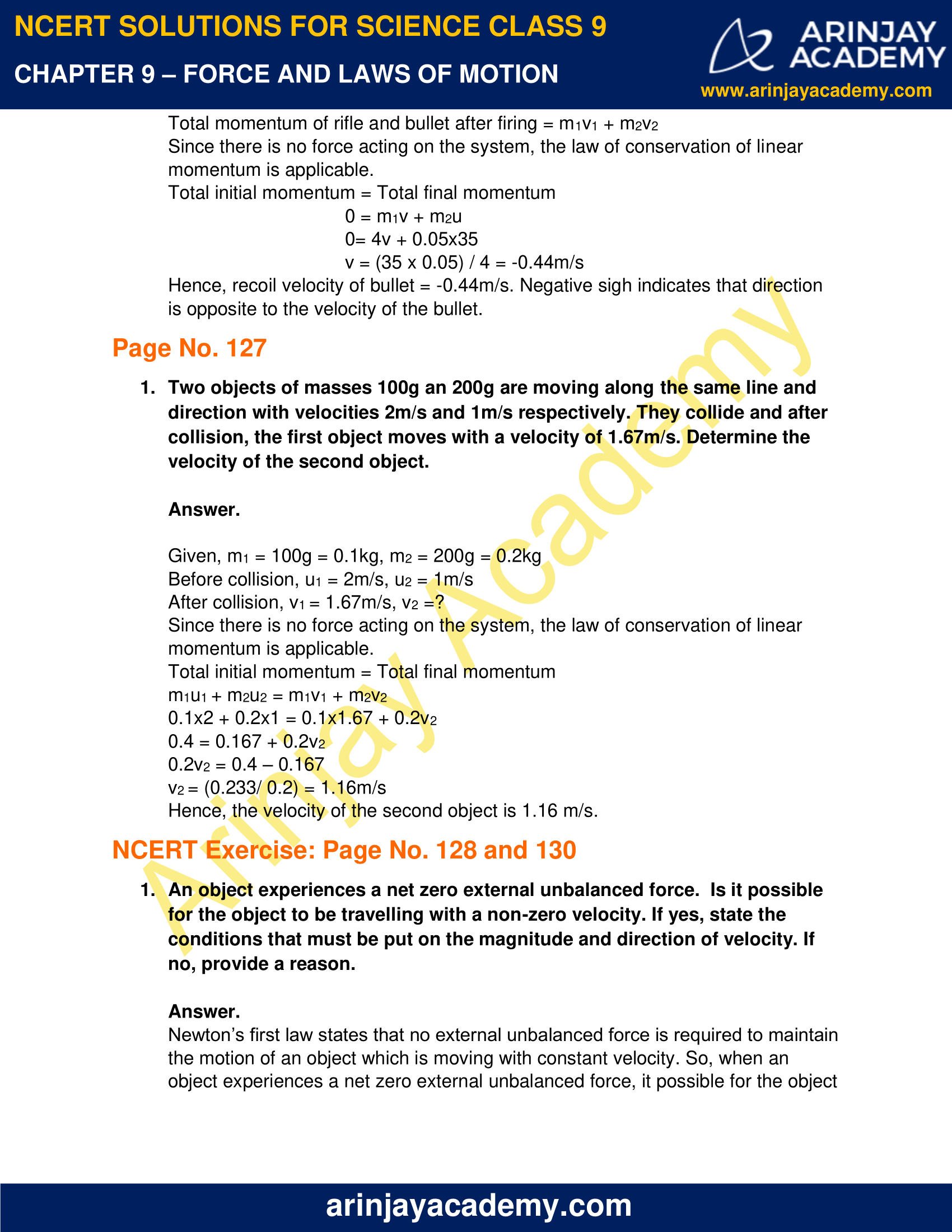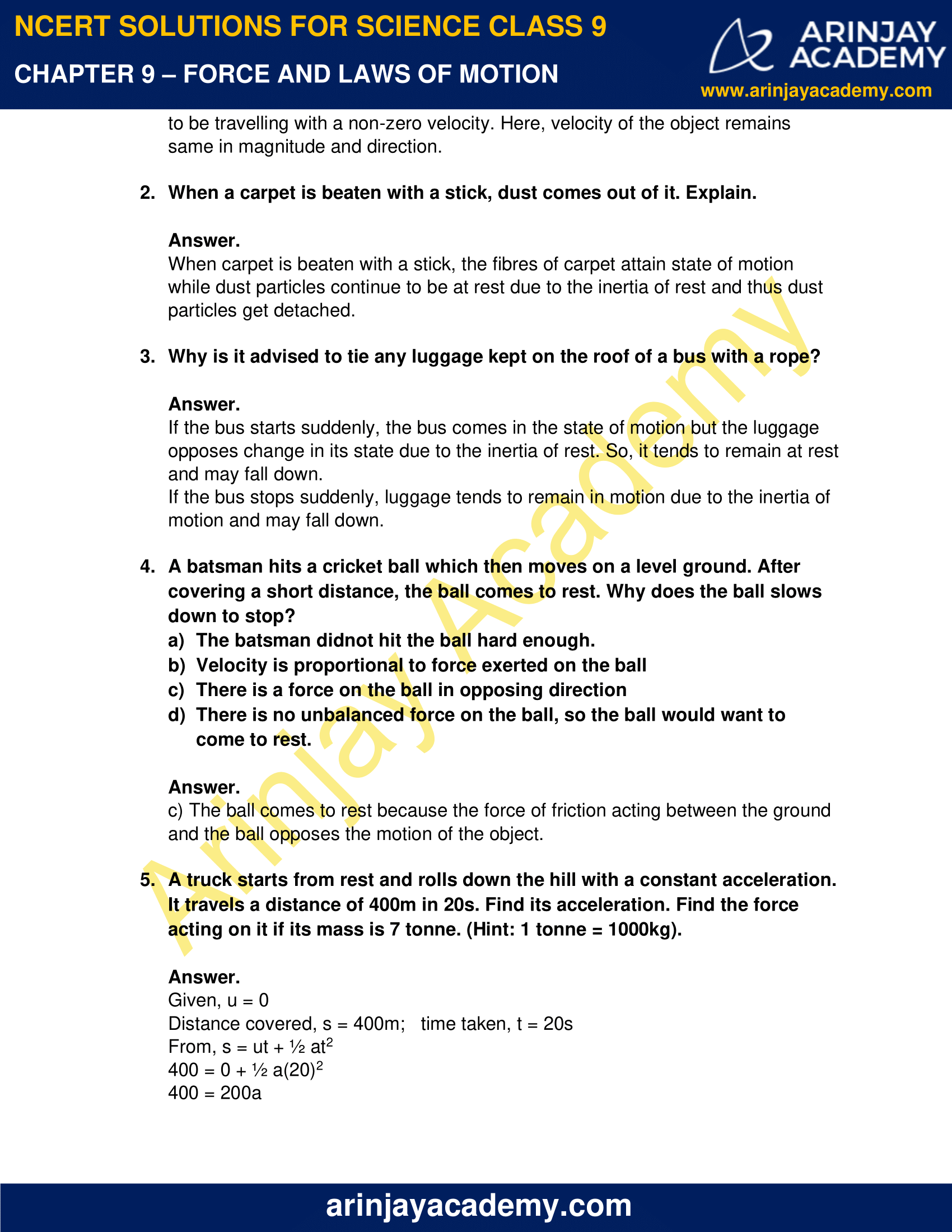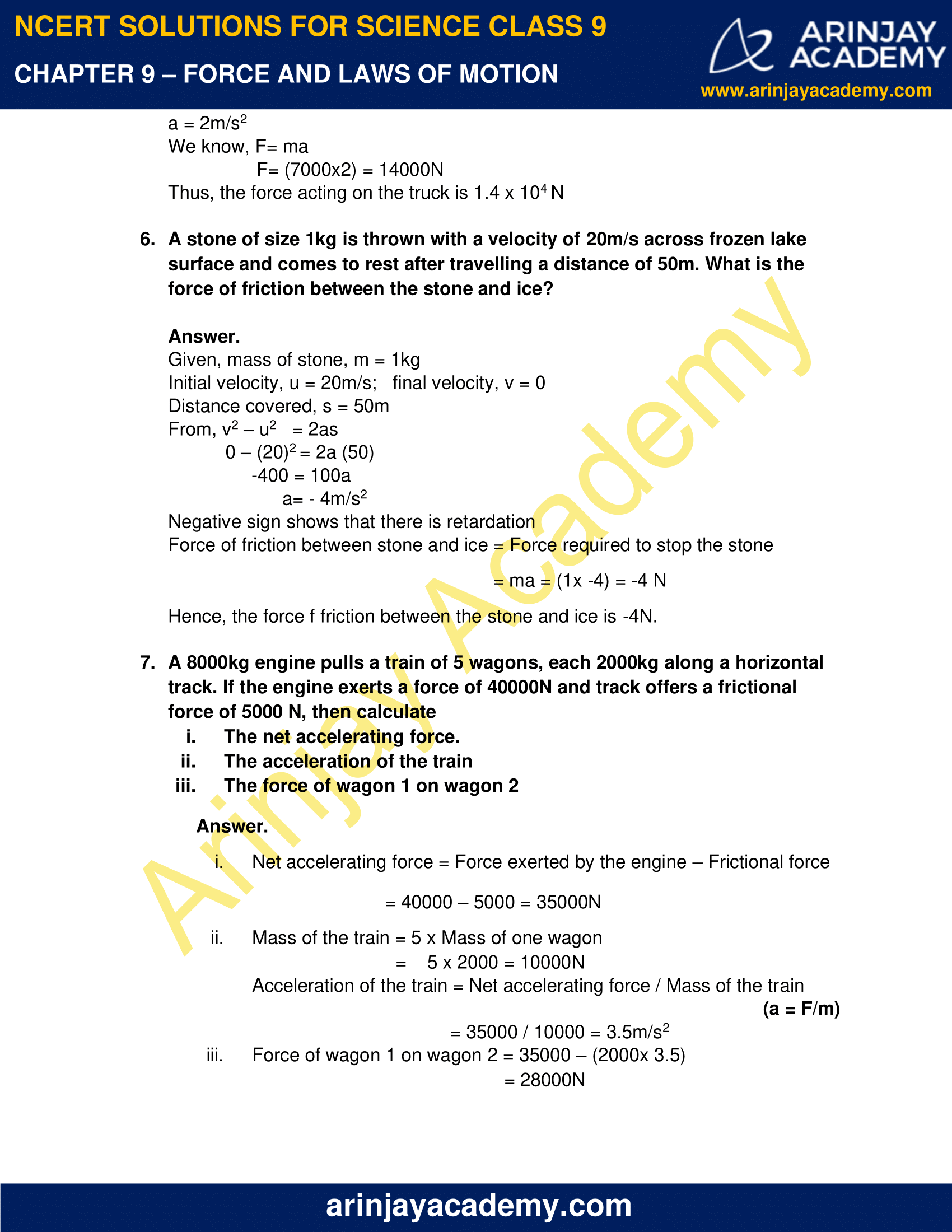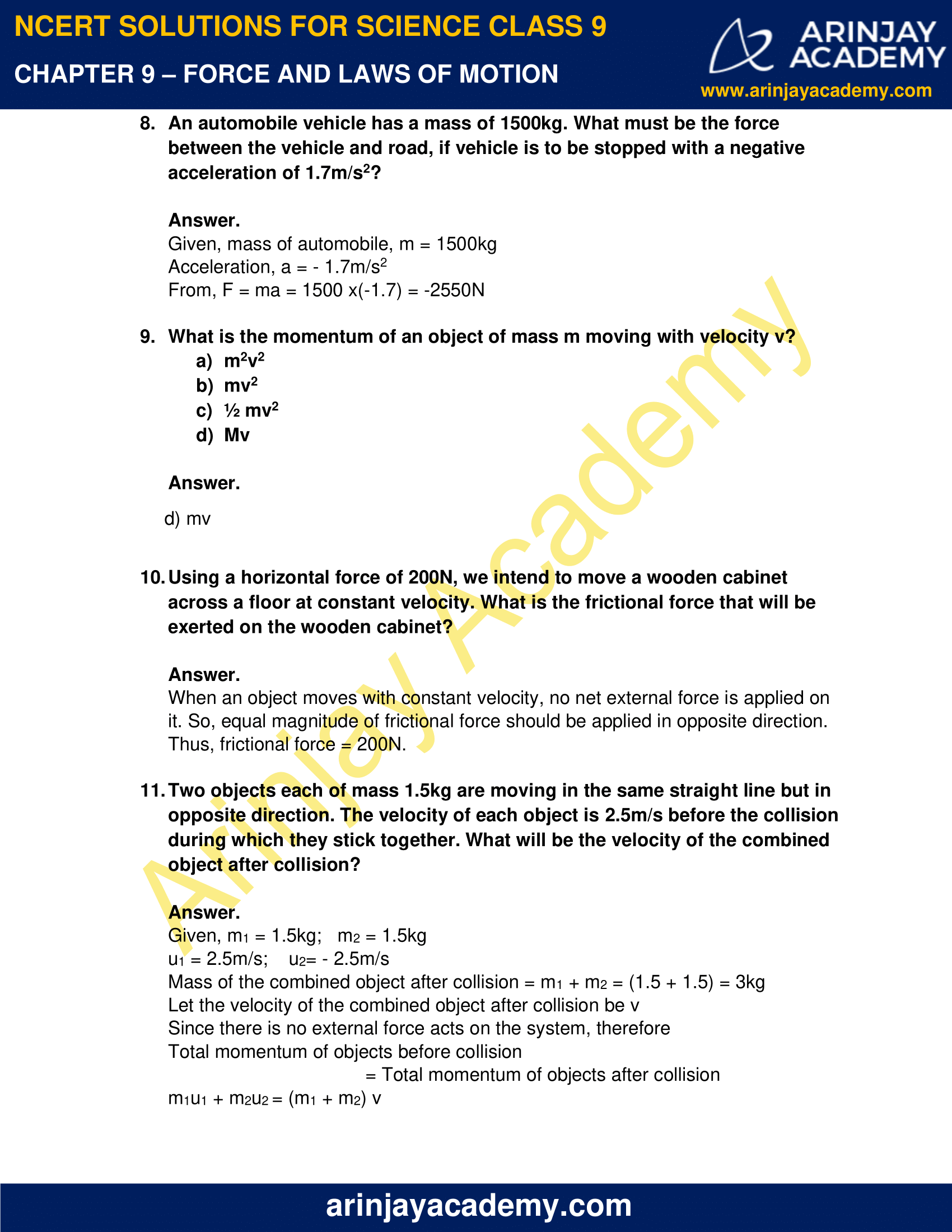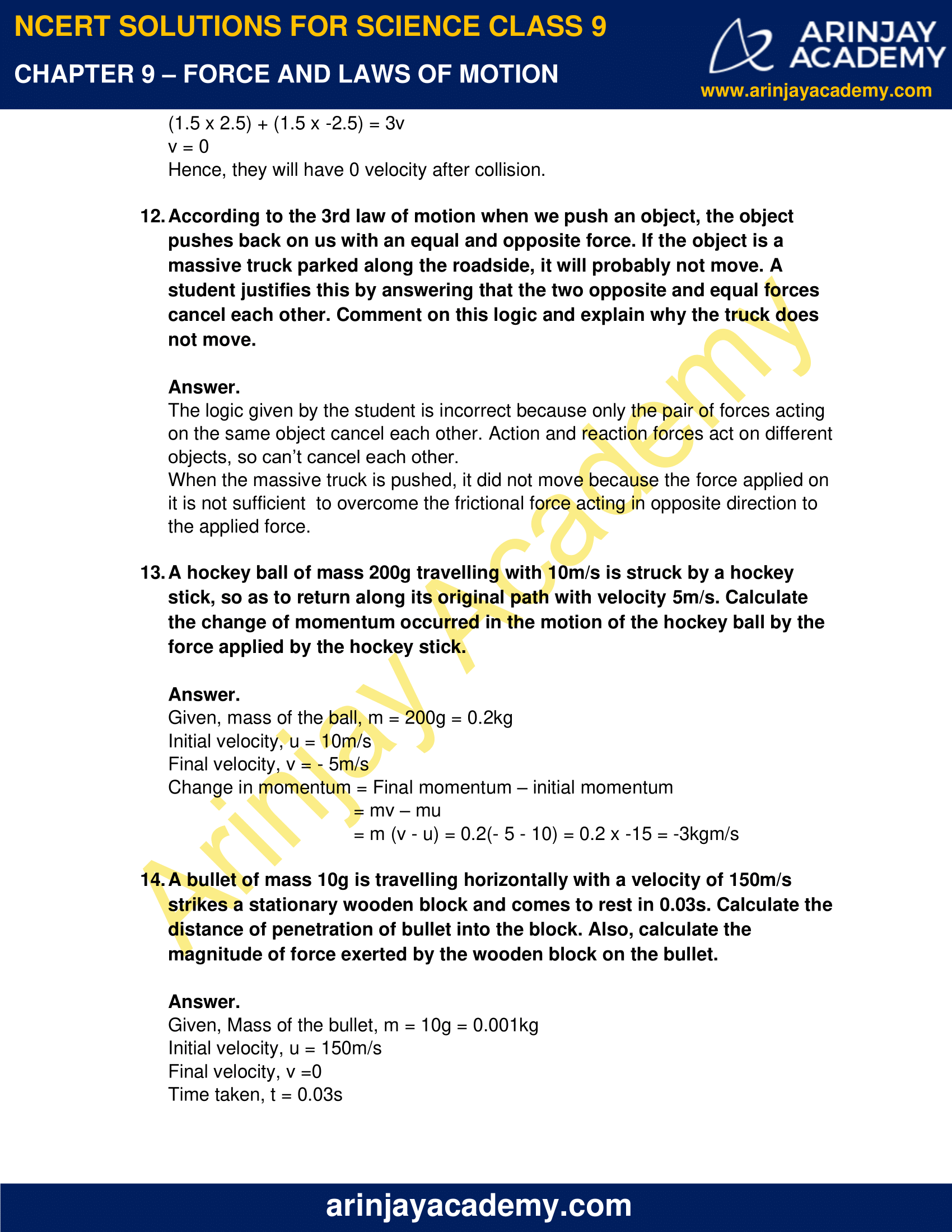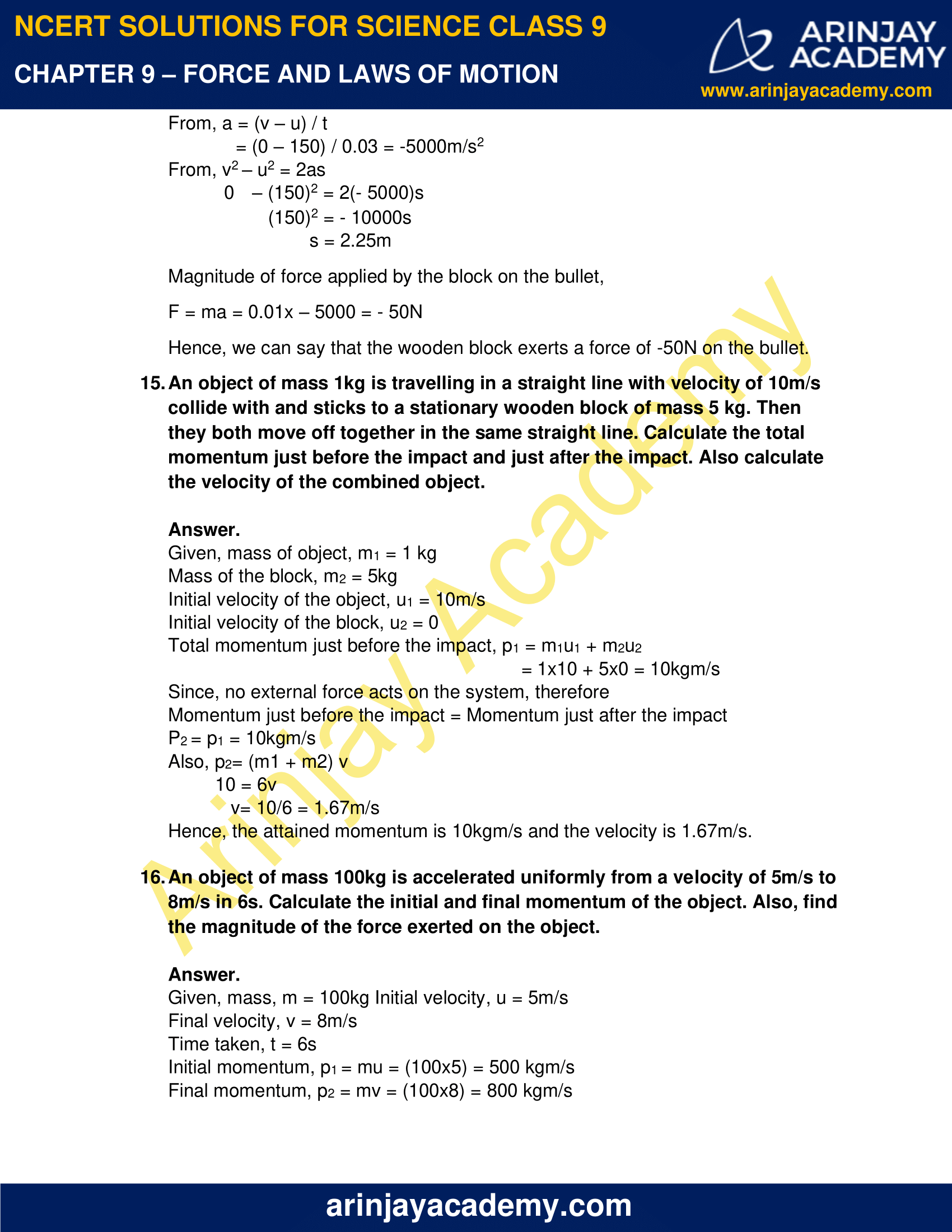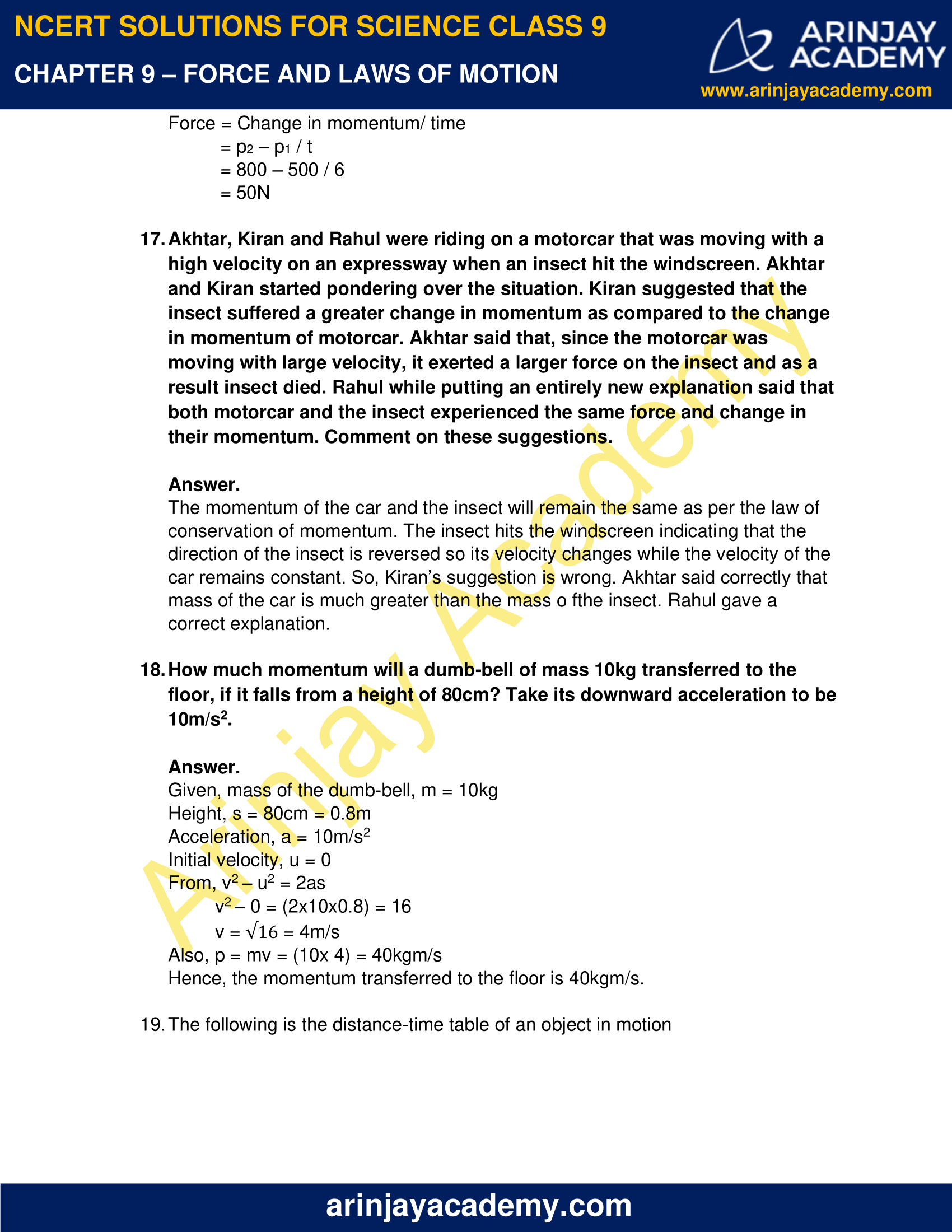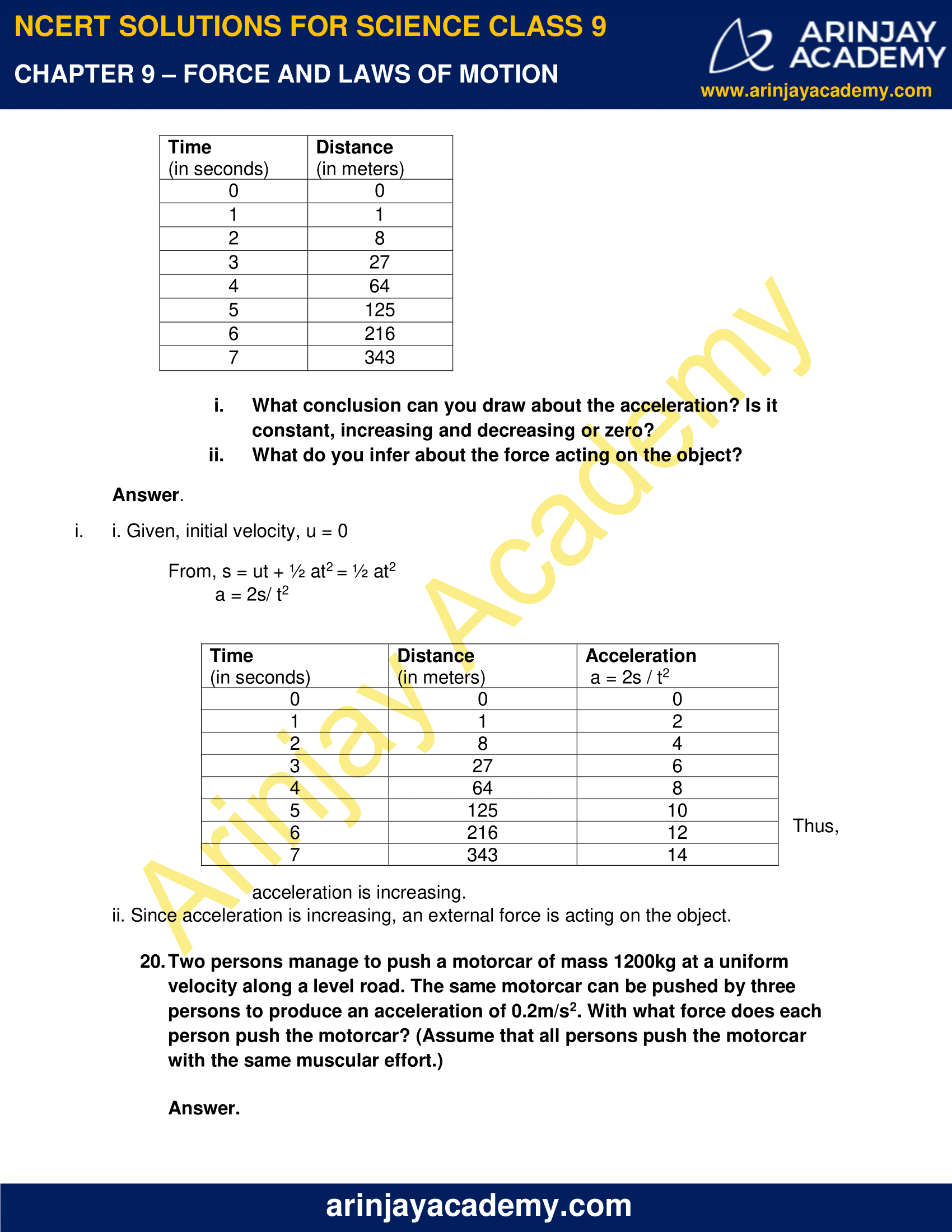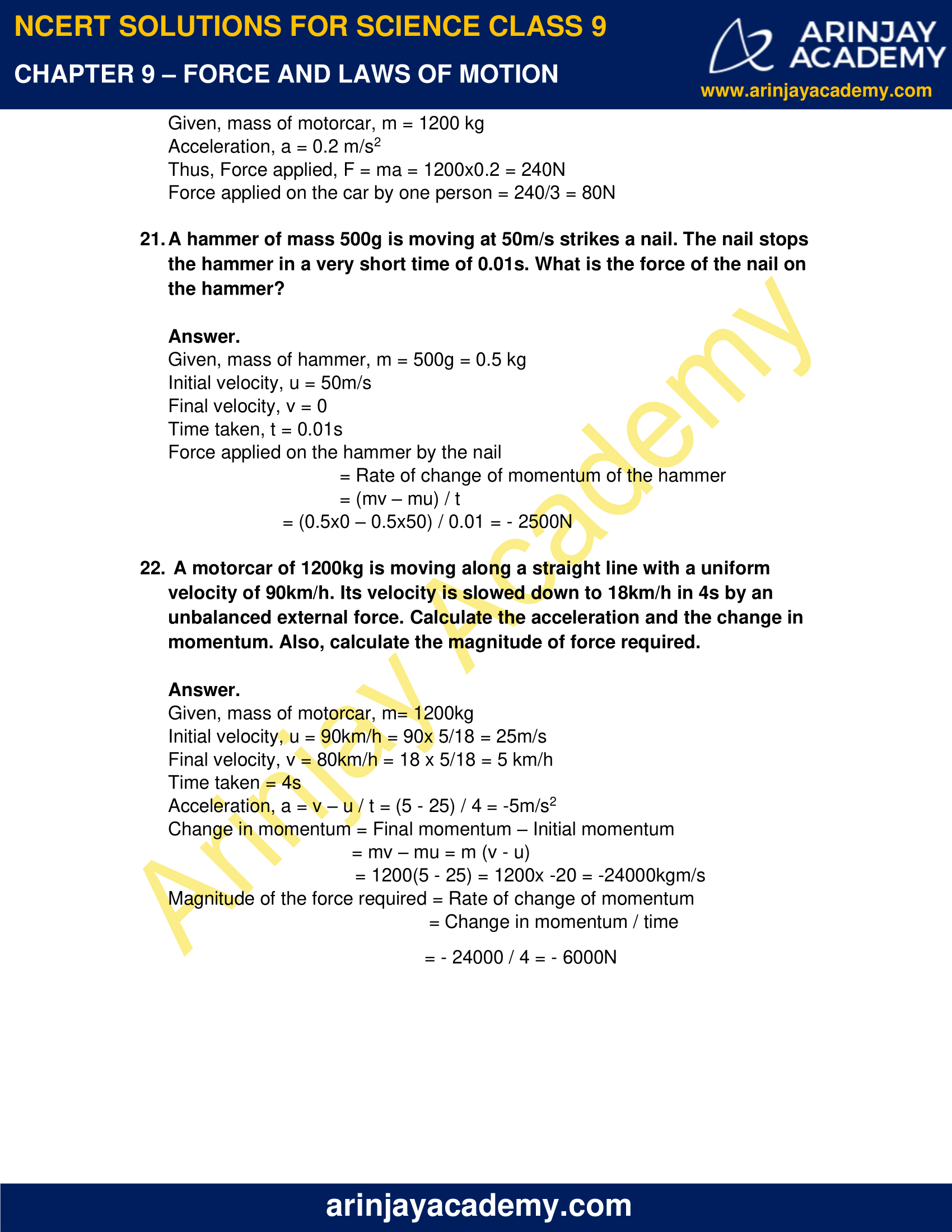### NCERT Solutions for Class 9 Science Chapter 9 – Force and Laws of Motion – NCERT Exercises

NCERT Solutions for Class 9 Science Chapter 9 – Force and Laws of Motion, Exercises includes all in text and exercise solved questions which helps you to understand the topic covered in Chapter 9 Force and Laws of Motion, in a better manner to help you to score good marks in your examinations.

### NCERT Solutions for Class 9 Science Chapter 9 – Force and Laws of Motion – Intext Questions: Page No. 118

1. Which of the following has more inertia?

i. A rubber ball and a stone of same size.
ii. A bicycle and a train.
iii. A five rupees coin and a one rupee coin.

Inertia of an object is proportional to its mass.

i. A stone of same size as a rubber ball will have greater mass, so stone will have more inertia.
ii. A train has greater mass than bicycle, so its inertia will be more.
iii. Between a five rupee coin and a one rupee coin, Five rupee coin has more mass, so will have greater inertia.

2. In the following example, try to identify the number of times the velocity of the ball changes.

A football player kicks a football to another player of his team who kicks the football towards the goal. The goalkeeper of opposite team collects the ball and kicks it towards a player of his own team. Also, identify the agent supplying the force in each case.

The velocity of the ball changes due to the application of force. The change may be in either in direction of velocity, or its magnitude or both. Here, number of times the velocity of the ball will change are

i. When first player kicks the football to another player of his team, he applies a force which will cause change in the velocity of the ball.
ii. When another player kicks the football towards the goal, he applies a force and so the velocity of the ball will change.
iii. As the goalkeeper collects the ball, its velocity will become zero. Here also, a force is applied to stop the ball.
iv. When the goalkeeper will pass the ball to another player of his own team, velocity will change again.

3. Explain why some leaves will get detached from a tree, if we rigorously shake its branch?

If we rigorously shake a branch of tree, some leaves get detached because the leaves possess inertia of rest and tend to remain in the same state when the position of branch changes.

4. Why do we fall in forward direction when a moving bus brakes to stop and fall backwards when it accelerates from rest?

We fall in forward direction when a moving bus brakes to stop because our body was in inertia of motion and opposes change in its state. Lower portion of the body being in contact with the bus, comes to rest and we tend to fall forward.

When a bus accelerates from rest, we had inertia of rest and our body resists change in its state. However, lower portion of the body starts moving with the bus, and the upper body fall backwards.

### Page No. 126

1. If action is always equal to reaction, then explain how a horse can pull a cart?

The horse pushes the ground in backward direction. As a reaction, ground exerts a reaction force on horse and cart to push them forward. When this force exceeds the frictional force between the wheels of cart and the ground, cart moves forward.

2. Explain why is it difficult for a fireman to hold a hose-pipe, which ejects large amount of water with high velocity.

It is difficult for a fireman to hold a hose-pipe, which ejects large amount of water with high velocity as the water exerts high pressure on the pipe. Due to the reaction of the forward force, a force is applied on the pipe in backward direction. Thus, it becomes difficult for the fire-man to keep the pipe at rest.

3. From a rifle of mass 4kg, a bullet of mass 50g is fired with an initial velocity of 35m/s. Calculate the initial recoil velocity of the rifle.

Given, Mass of rifle, m1 = 4kg

Mass of bullet, m2 = 50g = 0.05kg

Initial velocity of bullet, u = 35m/s

Let the recoil velocity of bullet be v

Since, both bullet and rifle are initially at rest,

Total momentum of rifle and bullet before firing = 0

Total momentum of rifle and bullet after firing = m1v1 + m2v2

Since there is no force acting on the system, the law of conservation of linear momentum is applicable.

Total initial momentum = Total final momentum

0 = m1v + m2u

0= 4v + 0.05×35

v = (35 x 0.05) / 4 = -0.44m/s

Hence, recoil velocity of bullet = -0.44m/s. Negative sigh indicates that direction is opposite to the velocity of the bullet.

### NCERT Solutions for Class 9 Science Chapter 9 – Force and Laws of Motion – Intext Questions – Page No. 127

1. Two objects of masses 100g an 200g are moving along the same line and direction with velocities 2m/s and 1m/s respectively. They collide and after collision, the first object moves with a velocity of 1.67m/s. Determine the velocity of the second object.

Given, m1 = 100g = 0.1kg, m2 = 200g = 0.2kg

Before collision, u1 = 2m/s, u2 = 1m/s

After collision, v1 = 1.67m/s, v2 =?

Since there is no force acting on the system, the law of conservation of linear momentum is applicable.

Total initial momentum = Total final momentum

m1u1 + m2u2 = m1v1 + m2v2

0.1×2 + 0.2×1 = 0.1×1.67 + 0.2v2

0.4 = 0.167 + 0.2v2

0.2v2 = 0.4 – 0.167

v2 = (0.233/ 0.2) = 1.16m/s

Hence, the velocity of the second object is 1.16 m/s.

### NCERT Solutions for Class 9 Science Chapter 9 – Force and Laws of Motion – NCERT Exercise: Page No. 128 and 130

1. An object experiences a net zero external unbalanced force. Is it possible for the object to be travelling with a non-zero velocity. If yes, state the conditions that must be put on the magnitude and direction of velocity. If no, provide a reason.

Newton’s first law states that no external unbalanced force is required to maintain the motion of an object which is moving with constant velocity. So, when an object experiences a net zero external unbalanced force, it possible for the object to be travelling with a non-zero velocity. Here, velocity of the object remains same in magnitude and direction.

2. When a carpet is beaten with a stick, dust comes out of it. Explain.

When carpet is beaten with a stick, the fibres of carpet attain state of motion while dust particles continue to be at rest due to the inertia of rest and thus dust particles get detached.

3. Why is it advised to tie any luggage kept on the roof of a bus with a rope?

If the bus starts suddenly, the bus comes in the state of motion but the luggage opposes change in its state due to the inertia of rest. So, it tends to remain at rest and may fall down.

If the bus stops suddenly, luggage tends to remain in motion due to the inertia of motion and may fall down.

4. A batsman hits a cricket ball which then moves on a level ground. After covering a short distance, the ball comes to rest. Why does the ball slows down to stop?

a) The batsman did not hit the ball hard enough.
b) Velocity is proportional to force exerted on the ball
c) There is a force on the ball in opposing direction
d) There is no unbalanced force on the ball, so the ball would want to come to rest.

c) The ball comes to rest because the force of friction acting between the ground and the ball opposes the motion of the object.

5. A truck starts from rest and rolls down the hill with a constant acceleration. It travels a distance of 400m in 20s. Find its acceleration. Find the force acting on it if its mass is 7 tonne. (Hint: 1 tonne = 1000kg).

Given, u = 0

Distance covered, s = 400m;   time taken, t = 20s

From, s = ut + ½ at2

400 = 0 + ½ a(20)2

400 = 200a

a = 2m/s2

We know, F= ma

F= (7000×2) = 14000N

Thus, the force acting on the truck is 1.4 x 104 N

6. A stone of size 1 kg is thrown with a velocity of 20m/s across frozen lake surface and comes to rest after travelling a distance of 50m. What is the force of friction between the stone and ice?

Given, mass of stone, m = 1kg

Initial velocity, u = 20m/s;

final velocity, v = 0

Distance covered, s = 50m

From, v2 – u2   = 2as

0 – (20)2 = 2a (50)

-400 = 100a

a= – 4m/s2

Negative sign shows that there is retardation

Force of friction between stone and ice = Force required to stop the stone

= ma = (1x -4) = -4 N

Hence, the force f friction between the stone and ice is -4N.

7. A 8000kg engine pulls a train of 5 wagons, each 2000kg along a horizontal track. If the engine exerts a force of 40000N and track offers a frictional force of 5000 N, then calculate

i. The net accelerating force.
ii. The acceleration of the train
iii. The force of wagon 1 on wagon 2

i. Net accelerating force = Force exerted by the engine – Frictional force

= 40000 – 5000 = 35000N

ii. Mass of the train = 5 x Mass of one wagon

=    5 x 2000 = 10000N

Acceleration of the train = Net accelerating force / Mass of the train

(a = F/m)

= 35000 / 10000 = 3.5m/s2

iii. Force of wagon 1 on wagon 2 = 35000 – (2000x 3.5)

= 28000N

8. An automobile vehicle has a mass of 1500kg. What must be the force between the vehicle and road, if vehicle is to be stopped with a negative acceleration of 1.7m/s2?

Given, mass of automobile, m = 1500kg

Acceleration, a = – 1.7m/s2

From, F = ma = 1500 x(-1.7) = -2550N

9. What is the momentum of an object of mass m moving with velocity v?

a) m2v2
b) mv2
c) ½ mv2
d) Mv

d) mv

10. Using a horizontal force of 200N, we intend to move a wooden cabinet across a floor at constant velocity. What is the frictional force that will be exerted on the wooden cabinet?

When an object moves with constant velocity, no net external force is applied on it. So, equal magnitude of frictional force should be applied in opposite direction.

Thus, frictional force = 200N.

11. Two objects each of mass 1.5kg are moving in the same straight line but in opposite direction. The velocity of each object is 2.5m/s before the collision during which they stick together. What will be the velocity of the combined object after collision?

Given, m1 = 1.5kg;
m2 = 1.5kg

u1 = 2.5m/s;
u2= – 2.5m/s

Mass of the combined object after collision = m1 + m2 = (1.5 + 1.5) = 3kg

Let the velocity of the combined object after collision be v

Since there is no external force acts on the system, therefore

Total momentum of objects before collision

= Total momentum of objects after collision

m1u1 + m2u2 = (m1 + m2) v

(1.5 x 2.5) + (1.5 x -2.5) = 3v

v = 0

Hence, they will have 0 velocity after collision.

12. According to the 3rd law of motion when we push an object, the object pushes back on us with an equal and opposite force. If the object is a massive truck parked along the roadside, it will probably not move. A student justifies this by answering that the two opposite and equal forces cancel each other. Comment on this logic and explain why the truck does not move.

The logic given by the student is incorrect because only the pair of forces acting on the same object cancel each other. Action and reaction forces act on different objects, so can’t cancel each other.

When the massive truck is pushed, it did not move because the force applied on it is not sufficient  to overcome the frictional force acting in opposite direction to the applied force.

13. A hockey ball of mass 200g travelling with 10m/s is struck by a hockey stick, so as to return along its original path with velocity 5m/s. Calculate the change of momentum occurred in the motion of the hockey ball by the force applied by the hockey stick.

Given, mass of the ball, m = 200g = 0.2kg

Initial velocity, u = 10m/s

Final velocity, v = – 5m/s

Change in momentum = Final momentum – initial momentum

= mv – mu

= m (v – u) = 0.2(- 5 – 10) = 0.2 x -15 = -3kgm/s

14. A bullet of mass 10g is travelling horizontally with a velocity of 150m/s strikes a stationary wooden block and comes to rest in 0.03s. Calculate the distance of penetration of bullet into the block. Also, calculate the magnitude of force exerted by the wooden block on the bullet.

Given, Mass of the bullet, m = 10g = 0.001kg

Initial velocity, u = 150m/s

Final velocity, v =0

Time taken, t = 0.03s

From, a = (v – u) / t

= (0 – 150) / 0.03 = -5000m/s2

From, v2 – u2 = 2as

0 – (150)2 = 2(- 5000)s

(150)2 = – 10000s

s = 2.25m

Magnitude of force applied by the block on the bullet,

F = ma = 0.01x – 5000 = – 50N

Hence, we can say that the wooden block exerts a force of -50N on the bullet.

15. An object of mass 1 kg is travelling in a straight line with velocity of 10m/s collide with and sticks to a stationary wooden block of mass 5 kg. Then they both move off together in the same straight line. Calculate the total momentum just before the impact and just after the impact. Also calculate the velocity of the combined object.

Given, mass of object, m1 = 1 kg

Mass of the block, m2 = 5kg

Initial velocity of the object, u1 = 10m/s

Initial velocity of the block, u2 = 0

Total momentum just before the impact, p1 = m1u1 + m2u2

= 1×10 + 5×0 = 10kgm/s

Since, no external force acts on the system, therefore

Momentum just before the impact = Momentum just after the impact

P2 = p1 = 10kgm/s

Also, p2= (m1 + m2) v

10 = 6v

v= 10/6 = 1.67m/s

Hence, the attained momentum is 10kgm/s and the velocity is 1.67m/s.

16. An object of mass 100kg is accelerated uniformly from a velocity of 5m/s to 8m/s in 6s. Calculate the initial and final momentum of the object. Also, find the magnitude of the force exerted on the object.

Given, mass, m = 100kg Initial velocity, u = 5m/s

Final velocity, v = 8m/s

Time taken, t = 6s

Initial momentum, p1 = mu = (100×5) = 500 kgm/s

Final momentum, p2 = mv = (100×8) = 800 kgm/s

Force = Change in momentum/ time

= p2 – p1 / t

= 800 – 500 / 6

= 50N

17. Akhtar, Kiran and Rahul were riding on a motorcar that was moving with a high velocity on an expressway when an insect hit the windscreen. Akhtar and Kiran started pondering over the situation. Kiran suggested that the insect suffered a greater change in momentum as compared to the change in momentum of motorcar. Akhtar said that, since the motorcar was moving with large velocity, it exerted a larger force on the insect and as a result insect died. Rahul while putting an entirely new explanation said that both motorcar and the insect experienced the same force and change in their momentum. Comment on these suggestions.

The momentum of the car and the insect will remain the same as per the law of conservation of momentum. The insect hits the windscreen indicating that the direction of the insect is reversed so its velocity changes while the velocity of the car remains constant. So, Kiran’s suggestion is wrong. Akhtar said correctly that mass of the car is much greater than the mass of the insect. Rahul gave a correct explanation.

18. How much momentum will a dumb-bell of mass 10kg transferred to the floor, if it falls from a height of 80cm? Take its downward acceleration to be 10m/s2.

Given, mass of the dumb-bell, m = 10kg

Height, s = 80 cm = 0.8m

Acceleration, a = 10 m/s2

Initial velocity, u = 0

From, v2 – u2 = 2as

v2 – 0 = (2x10x0.8) = 16

v =  = 4m/s

Also, p = mv = (10x 4) = 40kgm/s

Hence, the momentum transferred to the floor is 40kgm/s.

19. The following is the distance-time table of an object in motion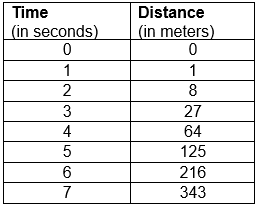i. What conclusion can you draw about the acceleration? Is it constant, increasing and decreasing or zero?

ii. What do you infer about the force acting on the object?

i. Given, initial velocity, u = 0

From, s = ut + ½ at2 = ½ at2

a = 2s/ t2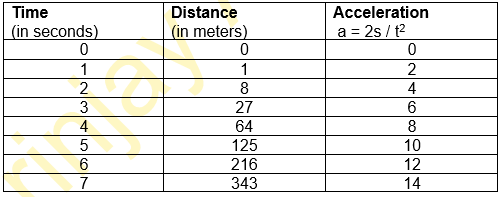Thus, acceleration is increasing.

ii. Since acceleration is increasing, an external force is acting on the object.

20. Two persons manage to push a motorcar of mass 1200kg at a uniform velocity along a level road. The same motorcar can be pushed by three persons to produce an acceleration of 0.2m/s2. With what force does each person push the motorcar? (Assume that all persons push the motorcar with the same muscular effort.)

Given, mass of motorcar, m = 1200 kg

Acceleration, a = 0.2 m/s2

Thus, Force applied, F = ma = 1200×0.2 = 240N

Force applied on the car by one person = 240/3 = 80N

21. A hammer of mass 500g is moving at 50m/s strikes a nail. The nail stops the hammer in a very short time of 0.01s. What is the force of the nail on the hammer?

Given, mass of hammer, m = 500g = 0.5 kg

Initial velocity, u = 50m/s

Final velocity, v = 0

Time taken, t = 0.01s

Force applied on the hammer by the nail

= Rate of change of momentum of the hammer

= (mv – mu) / t

= (0.5×0 – 0.5×50) / 0.01 = – 2500N

22. A motorcar of 1200 kg is moving along a straight line with a uniform velocity of 90km/h. Its velocity is slowed down to 18 km/h in 4s by an unbalanced external force. Calculate the acceleration and the change in momentum. Also, calculate the magnitude of force required.

Given, mass of motorcar, m= 1200kg

Initial velocity, u = 90km/h = 90x 5/18 = 25m/s

Final velocity, v = 80km/h = 18 x 5/18 = 5 km/h

Time taken = 4s

Acceleration, a = v – u / t = (5 – 25) / 4 = -5m/s2

Change in momentum = Final momentum – Initial momentum

= mv – mu = m (v – u)

= 1200(5 – 25) = 1200x -20 = -24000kgm/s

Magnitude of the force required = Rate of change of momentum

= Change in momentum / time

= – 24000 / 4 = – 6000N

### Topics Covered in Force and Laws of Motion Class 9 Science

1. Balanced and Unbalanced Forces
2. Newton’s Law of Motion
3. First Law of Motion
4. Inertia and Mass
5. Second Law of Motion
6. Third Law of Motion
7. Conservation of Momentum